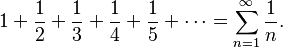# Divergent series facts for kids

Kids Encyclopedia Facts

In mathematics, a divergent series is an infinite series that is not convergent, meaning that the infinite sequence of the partial sums of the series does not have a finite limit.

If a series converges, the individual terms of the series must approach zero. Thus any series in which the individual terms do not approach zero diverges. However, convergence is a stronger condition: not all series whose terms approach zero converge. A counterexample is the harmonic series$1 + \frac{1}{2} + \frac{1}{3} + \frac{1}{4} + \frac{1}{5} + \cdots =\sum_{n=1}^\infty\frac{1}{n}.$

The divergence of the harmonic series was proven by the medieval mathematician Nicole Oresme.Divergent series Facts for Kids. Kiddle Encyclopedia.